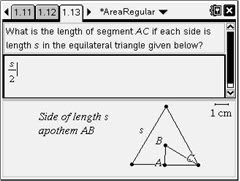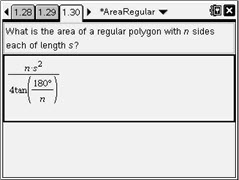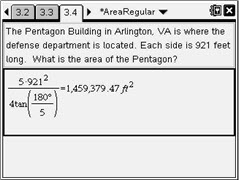# Activities

••• ##### Subject Area

• Math: Geometry: Quadrilaterals and Polygons

• ##### Author9-12

45 Minutes

• ##### Device
• TI-Nspire™
• TI-Nspire™ CAS
• ##### Software

TI-Nspire™
TI-Nspire™ CAS

2.1

## That Area Is So Regular#### Activity Overview

In this activity, students will investigate the formula for the area of regular polygons given the length of one side. Students will be asked inquiry questions to assist them in deriving the formula for a regular polygon with n= 3, 4, and 5 sides. They will then use the patterns they discovered to find the formula for the area of a regular polygon of n sides.

#### Key Steps

•In this activity, students will investigate the area of a regular polygon given the length of one side. Beginning with the formula for the area of a regular polygon (one half times the apothem times the perimeter), we will derive the formula for the area of a regular polygon in terms of the length of one side.

•Eventually, students will be asked to derive the formula for a regular polygon with n sides each of length s through inquiry questions that are identical to the questions for n = 3, 4, and 5.

•Finally, students are asked to solve real-world applications of the area of regular polygons. They are asked to find areas of a stop sign, a yield sign, the Pentagon Building, and the Sigil of Ameth.